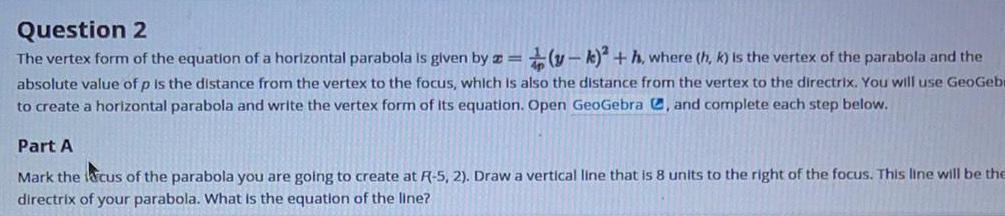Question:

# The vertex form of the equation of a horizontal parabola is

Last updated: 7/20/2022The vertex form of the equation of a horizontal parabola is given by z= 1/4p(y-k)2+h, where (h, k) is the vertex of the parabola and the absolute value of p is the distance from the vertex to the focus, which is also the distance from the vertex to the directrix. You will use GeoGebr to create a horizontal parabola and write the vertex form of its equation. Open GeoGebra , and complete each step below. Part A Mark the focus of the parabola you are going to create at R(-5, 2). Draw a vertical line that is 8 units to the right of the focus. This line will be the directrix of your parabola. What is the equation of the line?